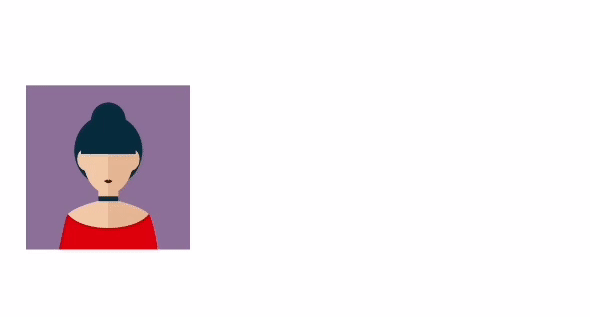# Android Custom View 102 (Part 19)In this post let’s take a deeper look at some of the more advanced uses of ObjectAnimator.

## KeyFrame

First example is the usage of KeyFrames. Here is the final result:Let’s look at the code

val imageView = findViewById<ImageView>(R.id.imageView)

/**
In total we want to move 300dp.
0% of time passed, it has moved 0dp.
30% of time passed, it has moved 100dp.
60% of time passed, it has moved 120dp.
100% of time passed, it has moved 300dp.
**/
val keyframe1 = Keyframe.ofFloat(0.0f, 0.0f)
val keyframe2 = Keyframe.ofFloat(0.3f, dp2px(100))
val keyframe3 = Keyframe.ofFloat(0.6f, dp2px(120))
val keyframe4 = Keyframe.ofFloat(1.0f, dp2px(300))

val holder = PropertyValuesHolder.ofKeyframe(
"translationX",
keyframe1,
keyframe2,
keyframe3,
keyframe4
)
val animator = ObjectAnimator.ofPropertyValuesHolder(imageView, holder)
animator.startDelay = 1000
animator.duration = 3000
animator.start()

## Custom TypeEvaluator

How do we animate a point like this:Well there are ways to do this, but let me introduce a more elegant way than what is probably in your head right now.

class PointView @JvmOverloads constructor(
context: Context, attrs: AttributeSet? = null, defStyleAttr: Int = 0
) : View(context, attrs, defStyleAttr) {

private val paint = Paint(Paint.ANTI_ALIAS_FLAG)

private var point: Point = Point(0, 0)

/**
* Functions for animation
*/
fun setPoint(point: Point) {
this.point = point
invalidate()
}
fun getPoint() = point

init {
paint.strokeWidth = dp2px(30)
paint.strokeCap = Paint.Cap.ROUND
paint.color = Color.BLUE
}

override fun onDraw(canvas: Canvas) {
super.onDraw(canvas)
canvas.drawPoint(point.x.toFloat(), point.y.toFloat(), paint)
}
}
val pointView = findViewById<PointView>(R.id.pointView)
val animator = ObjectAnimator.ofObject(
pointView,
"point",
object : TypeEvaluator<Point> {

override fun evaluate(
fraction: Float,
startValue: Point,
endValue: Point
): Point {
return Point(
(startValue.x + (endValue.x - startValue.x) * fraction).toInt(),
(startValue.y + (endValue.y - startValue.y) * fraction).toInt()
)
}
},
Point(0, 0),
Point(dp2px(200).toInt(), dp2px(200).toInt())
)